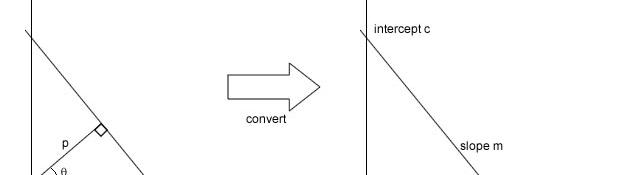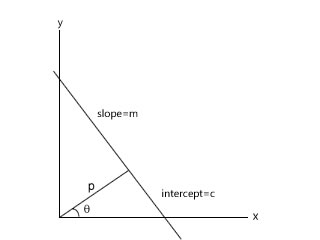## Converting lines from normal to slope-intercept form

Several algorithms return lines in the normal form (like The Hough transform) while other algorithms may require these lines in another format (like drawing a line on screen). The slop-intercept form of lines is widely known, and you can easily convert a line in slope-intercept form to other forms. So knowing how to efficiently do the conversion of parameters is a must! Here I'll show you one way to do this. But just in case, here's a brief review of the normal and slope-intercept forms.## The normal form

The normal form of a line uses two parameters: p and θ to describe the line. Here, p is the length of the perpendicular from the origin to the line. And θ is the angle between this perpendicular and the x-axis.

The line equation with these parameters is:

```p = x*cosθ + y*sinθ
```

## The slope-intercept form

This form of the line uses m and c as the parameters. m is the slope of the line (the tan of the angle between the line and the x-axis). And c is the position on the y-axis where the line intersects it.

The line equation with these parameters is:

```y = m*x + c
```

## The conversion

```p = x*cosθ + y*sinθ
```

Rearranging the equation, we get this:

```-x*cosθ + p =  y*sinθ
```

and dividing by sinθ we get:

```-x*cotθ + p*cosecθ =  y
```

or,

```y = -x*cotθ + p*cosecθ
```

and there you have the line in slope intercept form! Let me make it more explicit:

```y = (-cotθ)*x + (p*cosecθ)
```

-cotθ is the slope of the line, and p*cosecθ is the intercept.

Thus, we can summarize the conversion as:

```m = -cotθ c = p*cosecθ
```

Doing these calculations, you'll have your line in slope-intercept form instead of the normal form! Great!

#### Related posts#### 7 unique neural network architectures